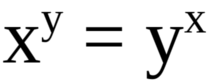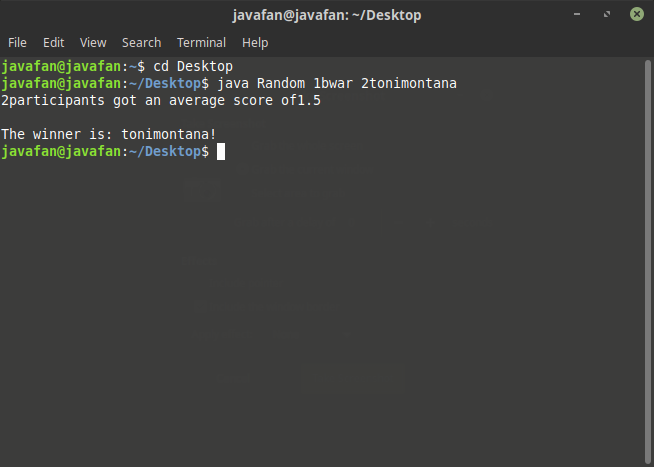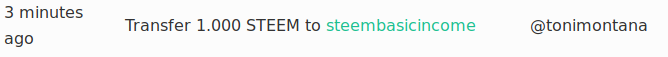# Mathematical SBI contest #3 Results and Solution of the Equation

in #puzzle3 years ago

The problem of the last contest was:There also was an additional condition which removed the obvious solution:
`x ≠ y`
To find the solution you could either start guessing around, or mess around with the equation and think a little:
`x^y = y^x` | y = x+n
`x^(x+n) = (x+n)^x`
`x^n = ((x+n)/x)^x`
`x^n = (1+n/x)^x`
`x^(n/x) = (1+n/x)` | z = n/x
`x^z = 1+z`
If z would be bigger than 1, the leftern term would grow to fast compared to the linear part, since the value of x has to be 2 or bigger.
So the rightern term can be at most 2.
Also we can assume here that x is bigger than 1, because the solutions are symmetrical. If (x₁|y₁) was a solution, (-x₁|-y₁) is also a solution.
If z is smaller than 1, The leftern term would get irration(because the root of an integer cannot be rational).
So the only solution is z = 1:
`x¹ = 1+1`

`x = 2`
`z = 1``y = x + 1*x = 4`
The other 3 integer solution follow directly from this, so all four integer solutions are:
`(2 | 4)`;
`(4 | 2)`;
`(-2 | -4)`;
`(-4 | -2)`;
As I stated in the rules I would give those better chances who got more solutions. I will do this by giving points for each correct solution(treating the solutions where x⇌y as one solution each). So you could get a total of 2 points.

Here a table of participants(sorted by time of entry):

Namesolutions foundscorechance of winning
@bwarx = 4; y = 211/3
@tonimontanax = 2; y = 4 \ x = -2; y = -422/3

I plugged the data into a self-written random picker:Congratulations @tonimontana. You won 1 SBI:Hopefully I will see you in the next contest will starts tomorrow.

Sort:

Oh damn, I was so close!

Come back next time, and you may win this.